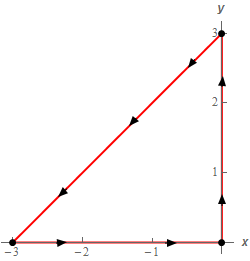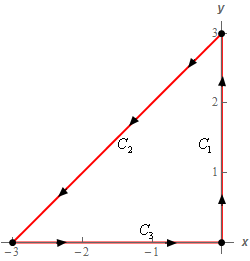Paul's Online Notes
Home / Calculus III / Line Integrals / Green's Theorem
Show Mobile Notice Show All Notes Hide All Notes
Mobile Notice
You appear to be on a device with a "narrow" screen width (i.e. you are probably on a mobile phone). Due to the nature of the mathematics on this site it is best views in landscape mode. If your device is not in landscape mode many of the equations will run off the side of your device (should be able to scroll to see them) and some of the menu items will be cut off due to the narrow screen width.

### Section 5-7 : Green's Theorem

5. Verify Green’s Theorem for $$\displaystyle \oint_{C}{{\left( {x{y^2} + {x^2}} \right)\,dx + \left( {4x - 1} \right)\,dy}}$$ where $$C$$ is shown below by (a) computing the line integral directly and (b) using Green’s Theorem to compute the line integral.Show All Solutions Hide All Solutions

a computing the line integral directly Show All Steps Hide All Steps
Start Solution

So, let’s start off the problem with labeling the curves as follows,Following the specified direction for each curve here are the parameterizations for each curve.

$${C_1}:\,\,\vec r\left( t \right) = \left\langle {0,t} \right\rangle \hspace{0.25in}0 \le t \le 3$$
$${C_2}:\,\,\vec r\left( t \right) = \left( {1 - t} \right)\left\langle {0,3} \right\rangle + t\left\langle { - 3,0} \right\rangle = \left\langle { - 3t,3 - 3t} \right\rangle \hspace{0.25in}0 \le t \le 1$$
$${C_3}:\,\,\vec r\left( t \right) = \left\langle {t,0} \right\rangle \hspace{0.25in} - 3 \le t \le 0$$

Show Step 2

Here is the line integral evaluated over each of these curves.

\begin{align*}\oint_{{{C_1}}}{{\left( {x{y^2} + {x^2}} \right)\,dx + \left( {4x - 1} \right)\,dy}} & = \int_{0}^{3}{{\left[ {\left( 0 \right){{\left( t \right)}^2} + {{\left( 0 \right)}^2}} \right]\left( 0 \right)\,dt}} + \int_{0}^{3}{{\left[ {4\left( 0 \right) - 1} \right]\left( 1 \right)\,dt}}\\ & = \int_{0}^{3}{{ - 1\,dt}} = \left. { - t} \right|_0^3 = \underline { - 3} \end{align*} \begin{align*}\oint_{{{C_2}}}{{\left( {x{y^2} + {x^2}} \right)\,dx + \left( {4x - 1} \right)\,dy}} & = \int_{0}^{1}{{\left[ {\left( { - 3t} \right){{\left( {3 - 3t} \right)}^2} + {{\left( { - 3t} \right)}^2}} \right]\left( { - 3} \right)\,dt}} + \int_{0}^{1}{{\left[ {4\left( { - 3t} \right) - 1} \right]\left( { - 3} \right)\,dt}}\\ & = \int_{0}^{1}{{81{t^3} - 189{t^2} + 81t\,dt}} + \int_{0}^{1}{{36t + 3\,dt}}\\ & = \int_{0}^{1}{{81{t^3} - 189{t^2} + 117t + 3\,dt}}\\ & = \left. {\left( {\frac{{81}}{4}{t^4} - 63{t^3} + \frac{{117}}{2}{t^2} + 3t} \right)} \right|_0^1 = \underline {\frac{{75}}{4}} \end{align*} \begin{align*}\oint_{{{C_3}}}{{\left( {x{y^2} + {x^2}} \right)\,dx + \left( {4x - 1} \right)\,dy}} & = \int_{{ - 3}}^{0}{{\left[ {\left( t \right){{\left( 0 \right)}^2} + {{\left( t \right)}^2}} \right]\left( 1 \right)\,dt}} + \int_{{ - 3}}^{0}{{\left[ {4\left( t \right) - 1} \right]\left( 0 \right)\,dt}}\\ & = \int_{{ - 3}}^{0}{{{t^2}\,dt}} = \left. {\frac{1}{3}{t^3}} \right|_{ - 3}^0 = \underline 9 \end{align*} Show Step 3

Now, all we need to do is add up the results from the previous step to get the value of the line integral over the full curve. This gives,

$\oint_{C}{{\left( {x{y^2} + {x^2}} \right)\,dx + \left( {4x - 1} \right)\,dy}} = \left( { - 3} \right) + \left( {\frac{{75}}{4}} \right) + \left( 9 \right) = \require{bbox} \bbox[2pt,border:1px solid black]{{\frac{{99}}{4}}}$

b using Green’s Theorem to compute the line integral Show All Steps Hide All Steps
Start Solution

Note that as the circle on the integral implies the curve is in the positive direction and so we can use Green’s Theorem on this integral.

From the integral we have,

$P = x{y^2} + {x^2}\hspace{0.25in}\hspace{0.25in}Q = 4x - 1$

Remember that $$P$$ is multiplied by $$x$$ and $$Q$$ is multiplied by $$y$$.

Show Step 2

Using Green’s Theorem the line integral becomes,

$\oint_{C}{{\left( {x{y^2} + {x^2}} \right)\,dx + \left( {4x - 1} \right)\,dy}} = \iint\limits_{D}{{4 - \left( {2xy} \right)\,dA}} = \iint\limits_{D}{{4 - 2xy\,dA}}$

$$D$$ is the region enclosed by the curve.

Show Step 3

We’ll leave it to you to verify that the equation of the line along the hypotenuse of the region is given by $$y = x + 3$$. Once we have this equation the region is then very easy to get limits for. They are,

$\begin{array}{c} - 3 \le x \le 0\\ 0 \le y \le x + 3\end{array}$ Show Step 4

Now all we need to do is evaluate the double integral. Here is the evaluation work.

\begin{align*}\oint_{C}{{\left( {x{y^2} + {x^2}} \right)\,dx + \left( {4x - 1} \right)\,dy}} & = \iint\limits_{D}{{4 - 2xy\,dA}}\\ & = \int_{{ - 3}}^{0}{{\int_{0}^{{x + 3}}{{4 - 2xy\,dy}}\,dx}}\\ & = \int_{{ - 3}}^{0}{{\left. {\left( {4y - x{y^2}} \right)} \right|_0^{x + 3}\,dx}}\\ & = \int_{{ - 3}}^{0}{{12 - 5x - 6{x^2} - {x^3}\,dx}}\\ & = \left. {\left( {12x - \frac{5}{2}{x^2} - 2{x^3} - \frac{1}{4}{x^4}} \right)} \right|_{ - 3}^0\\ & = \require{bbox} \bbox[2pt,border:1px solid black]{{\frac{{99}}{4}}}\end{align*}

So, we got the same answer after applying Green’s Theorem to the line integral as we got by integrating the line integral directly.